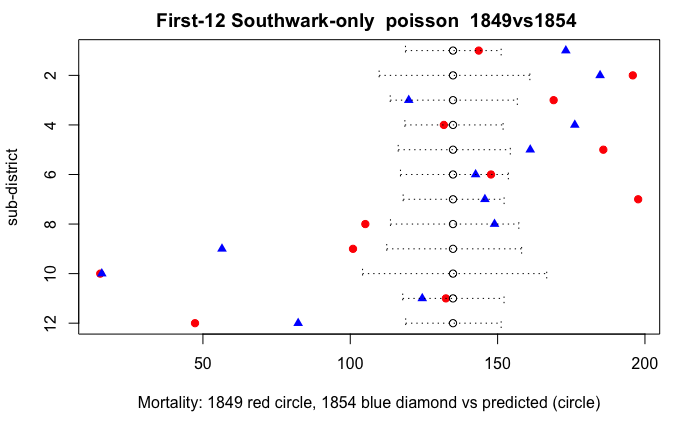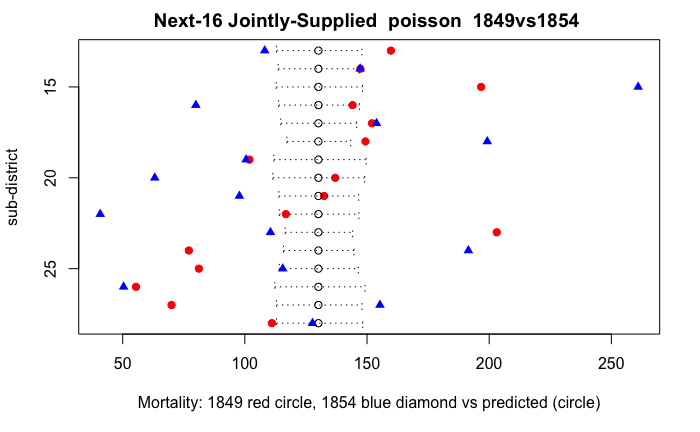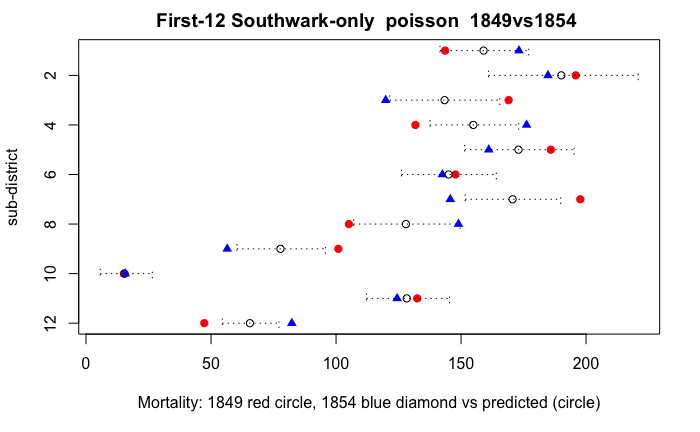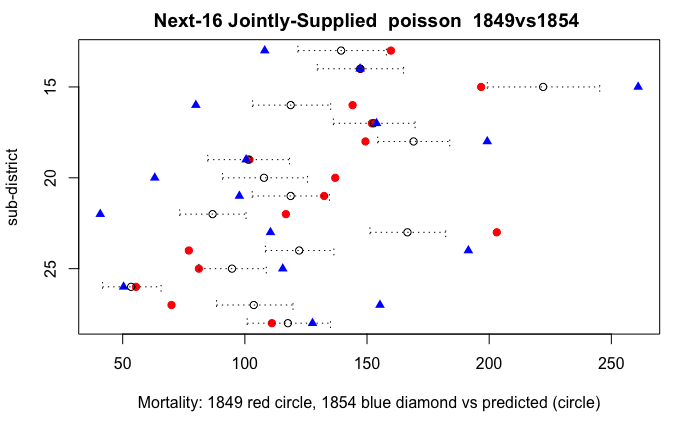# Difference in Differences Regressions - Error Analysis and Graphs for data from Snow 1855

### Introduction

This notebook discusses the count regressions (Poisson and Negative Binomial) for 1849 versus 1854 data that are run in the notebook Snow1855_DiDRegression1.Rmd.

The variation across sub-districts and within sub-districts across time (1849 versus 1854) are too large to be accounted for simply by random variation in counts with fixed mortality rates, as assumed for Poisson regression. We seem to be pushed towards the conclusion that the rates themselves are random, which would be consistent with Negative Binomial regression.

This notebook calculates and graphs the actual versus predicted rates under three assumptions:

• Poisson regression with rates the same across sub-districts
• Poisson regression but allowing rates to differ across sub-districts (fixed effects)
• Negative Binomial regression so that underlying Poisson rates are themsleves Gamma-distributed across sub-districts and time

For a brief introduction to Snow’s work, see:

• Snow’s original 1855 monograph (it is masterful): Snow, John. 1855. On the Mode of Communication of Cholera. 2nd ed. London: John Churchill. http://archive.org/details/b28985266.
• The best popular exposition I have found: Johnson, Steven. 2007. The Ghost Map: The Story of London’s Most Terrifying Epidemic–and How It Changed Science, Cities, and the Modern World. Reprint edition. New York: Riverhead Books.
• Another good popular version: Hempel, Sandra. 2007. The Strange Case of the Broad Street Pump: John Snow and the Mystery of Cholera. First edition. Berkeley: University of California Press.
• Tufte’s classic discussion of Snow’s mapping (a topic I don’t cover here): Tufte, Edward R. 1997. Visual Explanations: Images and Quantities, Evidence and Narrative. 1st edition. Graphics Press.
• Biography: Vinten-Johansen, Peter, Howard Brody, Nigel Paneth, Stephen Rachman, and Michael Russell Rip. 2003. Cholera, Chloroform and the Science of Medicine: A Life of John Snow. Oxford; New York: Oxford University Press. Linked on-line resources https://johnsnow.matrix.msu.edu/snowworks.php

This is an R Markdown Notebook. When you execute code within the notebook, the results appear beneath the code. The results are also saved in a self-contained html document with the suffix .nb.html. If you want pure r code (for example to run outside RStudio) you can easily extract code with the command knit(‘notebook.Rmd’,tangle=TRUE) which will save a file ‘notebook.R’ under your working directory.

Try executing the chunk below by clicking the Run button within the chunk or by placing your cursor inside it and pressing Cmd+Shift+Enter.

# Copyright (c) 2019, Thomas Coleman
#
#
# Results and discussion in "Causality in the Time of Cholera: John Snow as a Prototype
# for Causal Inference (Working Paper)" available at SSRN: https://papers.ssrn.com/abstract=3262234
rm(list=ls())    # starts a fresh workspace
#
library(knitr)
options(scipen=5)
# The following libraries are used for the Negative Binomial regression and the robust standard error analysis
#install.packages("sandwich")
#install.packages("lmtest")
library("MASS")
library("sandwich")
library("lmtest")
tablexii <- read.csv(file="Snow1855_TableXII.csv", header=TRUE, sep=",", skip=5,comment.char="#")

### “Worker” Functions for Plotting

The main object of this notebook is to plot mortality rates for sub-districts, say comparing 1849 versus 1854, with (approximate) error bars overlaid. The graphs are basically all the same with just different input data. So I have put together some simple functions that produce a standard format graph. These are in the file “SnowPlotFns.r” and then ’source’d into this notebook.

preperrdata Before graphing, however, we need to prepare the data

• Takes in fittedmodel - a regression that has been already run. From this it extracts the necessary parameters. Also single, a string which for “single” says that there is a single treatment effect.
• Calculates the 1849 and 1854 predicted counts and rates
• Calculates approximate 95% error bars around the predicted rates, based on whether the fitted model is Poisson or Negative Binomial
• Produces an adjusted 1854 predicted rate, adjusting for the 1854 time effect and treatment effect, so that it is comparable to the 1849 predicted rate (for purposes of plotting with error bars)

This function changes global data (the x1849 & x1854 dataframes) using the “<<-” instead of “<-” assignment. This is poor programming style but I could not find another easy way of doing what I wanted.

plot2_worker Plots actual vs predicted, with error bars around the predicted

plot2_worker Is a cover function which unpacks the actual versus predicted mean from the appropriate dataframe

plot3 Plots actual 1849, 1854 (adjusted for time & treatment effects), predicted, with error bars

plotcomp Plots actual 1849 versus 1854, with error bars around actual 1849

ploterrbars is NOT a function you should use - I use it to print out .pdf versions of the graphs I want to use

source('SnowPlotFns.r') 

### Create Regression Data

Now we create the we need for running count regressions, from Snow’s Tables XII and VIII. This is the same as in the notebook *Snow1855_DiDRegression1.Rmd.

x1 <- subset(tableviii,supplier == "SouthwarkVauxhall" | supplier == "SouthwarkVauxhall_Lambeth")
x1849 <- x1[c("subDistrict","pop1851","supplier","lambethdegree")]
x1 <- subset(tablexii,supplier == "SouthwarkVauxhall" | supplier == "SouthwarkVauxhall_Lambeth")
x1849$deaths <- x1$deaths1849
x1849$rate <- 10000 * x1$deaths1849 / x1849$pop1851 x1849$seq <- c(seq(1,length(x1849$deaths))) #x1849$dum1854 <- 0
xyear <- factor(c(rep(1849,28),rep(1854,28)))
x1849$year <- xyear[1:28] x1854 <- x1849 #x1849$lambethdegree <- "dirty"
x1854$deaths <- x1$deaths1854
x1854$rate <- 10000 * x1$deaths1854 / x1849$pop1851 x1854$seq <- c(seq(1,length(x1849$deaths))) #x1854$dum1854 <- 1
x1854\$year <- xyear[29:56]
regdata <- rbind(x1849,x1854)
regdata

### 1849 vs 1854 DiD, Poisson Regression

Now we are ready for running regressions and plotting. First, basic Poisson regression with single treatment effect:

$$ln(Rate) = ln(Count) - ln(Population) = \mu + \delta 54*I(54) + \gamma*I(joint) + \beta*I(54)*I(joint) + \epsilon$$

• an overall constant ($$\mu$$)
• a difference for 1854 ($$\delta54$$)
• a difference for joint “next 16” region ($$\gamma$$)
• an interaction for 1854 and joint ($$\beta$$)
# Poisson with single "Lambeth effect" and same rate for all sub-districts (no sub-district fixed effects)
pois1single <- glm(deaths ~ supplier * year
+ offset(log(pop1851)), family=poisson, data=regdata)
summary(pois1single)

Call:
glm(formula = deaths ~ supplier * year + offset(log(pop1851)),
family = poisson, data = regdata)

Deviance Residuals:
Min        1Q    Median        3Q       Max
-12.1542   -3.0953    0.0306    2.7398   10.2518

Coefficients:
Estimate Std. Error  z value Pr(>|z|)
(Intercept)                                -4.30610    0.02103 -204.755  < 2e-16 ***
supplierSouthwarkVauxhall_Lambeth          -0.03593    0.02643   -1.359  0.17400
year1854                                    0.08354    0.02914    2.867  0.00414 **
supplierSouthwarkVauxhall_Lambeth:year1854 -0.51088    0.03870  -13.201  < 2e-16 ***
---
Signif. codes:  0 ‘***’ 0.001 ‘**’ 0.01 ‘*’ 0.05 ‘.’ 0.1 ‘ ’ 1

(Dispersion parameter for poisson family taken to be 1)

Null deviance: 2032.6  on 55  degrees of freedom
Residual deviance: 1541.6  on 52  degrees of freedom
AIC: 1937.4

Number of Fisher Scoring iterations: 4

This regression calculates the same parameter as the simple table (-0.5109, from notebooks Snow1855_DiDRegression1.Rmd or Snow1855_SimpleDID_QRCT.Rmd). The Poisson regression says the z value is -13.2 but this is in fact a huge over-estimate. Graphing the actual versus fitted mortality rates by sub-district help to show why.

xfamily <- preperrdata(pois1single,"single")  # this function modifies global data
plot3(x1849,x1854,"SouthwarkVauxhall",paste("First-12 Southwark-only ",xfamily," 1849vs1854 "))plot3(x1849,x1854,"SouthwarkVauxhall_Lambeth",paste("Next-16 Jointly-Supplied ",xfamily," 1849vs1854 "))The empty circles are the fitted with error bars; red circles are 1849; blue diamonds are 1854 (adjusted for year and treatment effects to be comparable with 1849). The first graph shows the “first-12” Southwark-onl sub-districs and the second the “next-12” jointly-supplied sub-districts. (The sequence numbers match the sequence or IDs in Table XII or Table VIII.) The error bars are estimated 95% limits assuming that the counts are Poisson-distributed (which is for all practical purposes the same as assuming counts are Binomial, generated from a Bernoulli process). Note that smaller sub-districts, such as Putney (ID 10, population 5,280) have wider error bars.

The problem is obvious from these graphs: the observed rates are almost all outside the error bars. There is simply too much variation, both across sub-districts and within sub-districts, to be consistent with a Poisson process with all sub-districts having the same mortality.

The regression statistic we need to use is the “Residual Deviance” which essentially measures the sum-of-squared differences between actual and predicted - larger when the data fit less well. This will be approximately chi-squared distributed, with 52 degrees of freedom in this case. The value is 1541.6 which is very large - the 5% right-tail quantile for a chi-squared with 52-degrees of freedom is 69.8 - a value larger than this will only be observed with 5% probability. A value of 1541.6 is far out in the right tail, with miniscule probability of being observed. In sum, it would be exceedinlgy unlikely to observe a Residual Deviance of 1541.6 if the data were Poisson-distributed - we can reject the hypothesis that the observed counts are generated by a Poisson process.

We have to abandon the assumption that rates are Poisson with a constant rate for all sub-districts.

$$ln(Rate) = ln(Count) - ln(Population) = \mu + \delta 54*I(54) + \gamma*I(joint) + \beta*I(54)*I(joint) + \epsilon$$

One direction we can go is to allow the mean rate $$\mu$$ to vary by sub-district: sub-district fixed effects. The other is to generalize the error process and allow $$\epsilon$$ to be other than Poisson-distributed: say Negative Binomial

### 1849 vs 1854 DiD, Poisson Regression with Fixed effects

So our first generalization is sub-district fixed effects. Our data has a “subDistric” factor that we can use in our regression:

# Poisson with single "Lambeth effect" and different rates by sub-district (fixed effects)
pois2single <- glm(deaths ~ subDistrict + supplier * year
+ offset(log(pop1851)), family=poisson, data=regdata)
summary(pois2single)

Call:
glm(formula = deaths ~ subDistrict + supplier * year + offset(log(pop1851)),
family = poisson, data = regdata)

Deviance Residuals:
Min       1Q   Median       3Q      Max
-6.8307  -2.1772  -0.0007   2.1558   7.2691

Coefficients: (1 not defined because of singularities)
Estimate Std. Error z value Pr(>|z|)
(Intercept)                                -4.358711   0.061453 -70.928  < 2e-16 ***
subDistrictBorough Road                     0.551368   0.074805   7.371 1.70e-13 ***
subDistrictBrixton                         -0.873070   0.107840  -8.096 5.68e-16 ***
subDistrictCamberwell                       0.002546   0.075176   0.034 0.972980
subDistrictChristchurch, Southwark          0.085657   0.081164   1.055 0.291260
subDistrictClapham                         -0.210516   0.086382  -2.437 0.014808 *
subDistrictKennington (1st)                -0.045641   0.076931  -0.593 0.552997
subDistrictKennington (2nd)                -0.300608   0.085249  -3.526 0.000422 ***
subDistrictKent Road                        0.140521   0.078391   1.793 0.073042 .
subDistrictLambeth Church (1st)            -0.388067   0.087552  -4.432 9.32e-06 ***
subDistrictLambeth Church (2nd)             0.263607   0.072349   3.644 0.000269 ***
subDistrictLeather Market                   0.125367   0.075538   1.660 0.096981 .
subDistrictLondon Road                     -0.074462   0.082065  -0.907 0.364220
subDistrictPeckham                         -0.668876   0.085472  -7.826 5.05e-15 ***
subDistrictPutney                          -2.115547   0.249739  -8.471  < 2e-16 ***
subDistrictRotherhithe                      0.287736   0.071578   4.020 5.82e-05 ***
subDistrictSt. George, Camberwell          -0.084183   0.084406  -0.997 0.318588
subDistrictSt. James, Bermondsey            0.191154   0.071991   2.655 0.007925 **
subDistrictSt. John, Horsleydown            0.114013   0.080544   1.416 0.156908
subDistrictSt. Mary Magdalen                0.301424   0.074392   4.052 5.08e-05 ***
subDistrictSt. Mary, Newington             -0.233003   0.090182  -2.584 0.009775 **
subDistrictSt. Olave, Southwark             0.395903   0.081797   4.840 1.30e-06 ***
subDistrictSt. Peter, Walworth              0.278504   0.071250   3.909 9.28e-05 ***
subDistrictSt. Saviour, Southwark           0.217198   0.071240   3.049 0.002297 **
subDistrictTrinity, Newington               0.177119   0.075970   2.331 0.019731 *
subDistrictWandsworth                      -0.497886   0.099782  -4.990 6.05e-07 ***
subDistrictWaterloo Road (1st)             -0.171047   0.088666  -1.929 0.053716 .
subDistrictWaterloo Road (2nd)             -0.074593   0.081580  -0.914 0.360532
supplierSouthwarkVauxhall_Lambeth                 NA         NA      NA       NA
year1854                                    0.083541   0.029140   2.867 0.004145 **
supplierSouthwarkVauxhall_Lambeth:year1854 -0.510882   0.038702 -13.201  < 2e-16 ***
---
Signif. codes:  0 ‘***’ 0.001 ‘**’ 0.01 ‘*’ 0.05 ‘.’ 0.1 ‘ ’ 1

(Dispersion parameter for poisson family taken to be 1)

Null deviance: 2032.61  on 55  degrees of freedom
Residual deviance:  456.78  on 26  degrees of freedom
AIC: 904.6

Number of Fisher Scoring iterations: 4

We can plot this with our plot3 function to examine the actual versus predicted.

xfamily <- preperrdata(pois2single,"single")  # this function modifies global data
plot3(x1849,x1854,"SouthwarkVauxhall",paste("First-12 Southwark-only ",xfamily," 1849vs1854 "))plot3(x1849,x1854,"SouthwarkVauxhall_Lambeth",paste("Next-16 Jointly-Supplied ",xfamily," 1849vs1854 "))Now the individual sub-districts all have different rates. But still the data do not fit well. There are too many of the red circles or blue diamonds outside the error bars - there should only be 5% or roughly 3 out of 56. And the residual deviance, 456.8 is still too large compared with the chi-squared (with 26 df) of 38.9

### 1849 vs 1854 DiD, Negative Binomial Regression

Instead of allowing each sub-district to have its own, fixed, rate, we are going to take another direction. For our regression equation:

$$ln(Rate) = ln(Count) - ln(Population) = \mu + \delta 54*I(54) + \gamma*I(joint) + \beta*I(54)*I(joint) + \epsilon$$

we will allow the error $$\epsilon$$ to have a more general distribution, Negative Binomial in this case. A Negative Binomial distribution is actually a mixture of Poisson distributions but with the underling Poisson rate itself now random, chosen from a Gamma distribution. (See my working paper at https://papers.ssrn.com/abstract=3262234 and references there for more details.)

# Negative Binomial with single "Lambeth effect"
nb1single <- glm.nb(deaths ~ supplier * year
+ offset(log(pop1851)), data=regdata)
summary(nb1single)

Call:
glm.nb(formula = deaths ~ supplier * year + offset(log(pop1851)),
data = regdata, init.theta = 4.956204025, link = log)

Deviance Residuals:
Min       1Q   Median       3Q      Max
-3.2718  -0.5282   0.0652   0.4958   1.8326

Coefficients:
Estimate Std. Error z value Pr(>|z|)
(Intercept)                                -4.33237    0.13169 -32.898   <2e-16 ***
supplierSouthwarkVauxhall_Lambeth          -0.03182    0.17386  -0.183   0.8548
year1854                                    0.05734    0.18616   0.308   0.7581
supplierSouthwarkVauxhall_Lambeth:year1854 -0.50027    0.24612  -2.033   0.0421 *
---
Signif. codes:  0 ‘***’ 0.001 ‘**’ 0.01 ‘*’ 0.05 ‘.’ 0.1 ‘ ’ 1

(Dispersion parameter for Negative Binomial(4.9562) family taken to be 1)

Null deviance: 71.825  on 55  degrees of freedom
Residual deviance: 59.764  on 52  degrees of freedom
AIC: 657.19

Number of Fisher Scoring iterations: 1

Theta:  4.956
Std. Err.:  0.973

2 x log-likelihood:  -647.185 

One thing we want to immediately note is the Residual Deviance is only 59.8 which is well below the 5% right-tail quantile of 69.8, meaning that such a value would not be unusual for Negative Binomial counts. (In fact the right-tail probability is 0.214.)

xfamily <- preperrdata(nb1single,"single")  # this function modifies global data
plot3(x1849,x1854,"SouthwarkVauxhall",paste("First-12 Southwark-only ",xfamily," 1849vs1854 "))